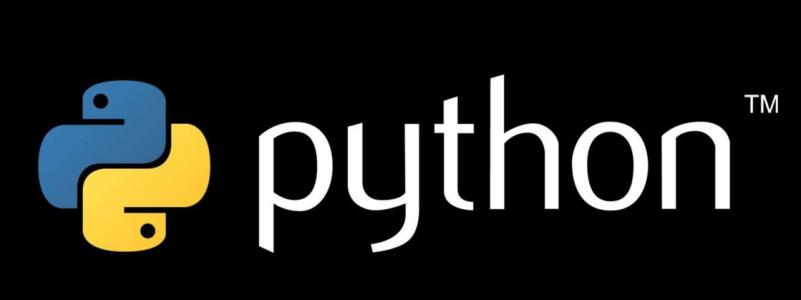Jquery中文网 www.jquerycn.cn
Jquery中文网 >  Python编程  >  Python入门  >  正文 深入理解Python的流程控制

# 深入理解Python的流程控制

jquery中文网为您提供深入理解Python的流程控制等资源，欢迎您收藏本站，我们将为您提供最新的深入理解Python的流程控制资源if 语句

```>>> x = int(input("Please enter an integer: "))
>>> if x < 0:
...     x = 0
...     print('Negative changed to zero')
... elif x == 0:
...     print('Zero')
... elif x == 1:
...     print('Single')
... else:
...     print('More')
...```

for 语句

Python 中 for 语句有点不同于 C 和 Pascal 中的 for 语句。Python 的 for 语句按照项目在序列中出现的顺序迭代任何序列（列表或字符串），而不是总是迭代数学的算术级数（如 Pascal 中），或者让用户能够定义迭代步骤和停止条件（如 C），例如（没有双关语）：

```>>> # 测量字符串：
... words = ['cat', 'window', 'defenestrate']
>>> for w in words:
...     print(w, len(w))
...
cat 3
window 6
defenestrate 12```

```>>> for w in words[:]:  # 循环遍历整个列表的切片副本。
...     if len(w) > 6:
...         words.insert(0, w)
...
>>> words
['defenestrate', 'cat', 'window', 'defenestrate']```

range() 函数

```>>> for i in range(5):
...     print(i)
...
0
1
2
3
4```

```range(5, 10)
5, 6, 7, 8, 9

range(0, 10, 3)
0, 3, 6, 9

range(-10, -100, -30)
-10, -40, -70```

```>>> a = ['Mary', 'had', 'a', 'little', 'lamb']
>>> for i in range(len(a)):
...     print(i, a[i])
...
0 Mary
2 a
3 little
4 lamb```

```>>> print(range(10))
range(0, 10)```

```>>> list(range(5))
[0, 1, 2, 3, 4]```

break 和 continue 语句，以及循环上的 else 子句

break 语句，类似于 C ，会打破 for 或 while 循环的最内层。

```>>> for n in range(2, 10):
...     for x in range(2, n):
...         if n % x == 0:
...             print(n, 'equals', x, '*', n//x)
...             break
...     else:
...         # 没有找到一个因数导致的循环失败
...         print(n, 'is a prime number')
...
2 is a prime number
3 is a prime number
4 equals 2 * 2
5 is a prime number
6 equals 2 * 3
7 is a prime number
8 equals 2 * 4
9 equals 3 * 3```

（是的，这是正确的代码。密切关注： for 循环的 else 子句，不是 if 语句。）

continue 语句，也是从 C 借来的，用于继续循环的下一次迭代：

```>>> for num in range(2, 10):
...     if num % 2 == 0:
...         print("Found an even number", num)
...         continue
...     print("Found a number", num)
Found an even number 2
Found a number 3
Found an even number 4
Found a number 5
Found an even number 6
Found a number 7
Found an even number 8
Found a number 9```

pass 语句

pass 语句什么也不做。它可以用于语法上需要，但程序不需要做什么的时候。例如：

```>>> while True:
...     pass  # 等待键盘中断（Ctrl C）
...```

```>>> class MyEmptyClass:
...     pass
...```
[]